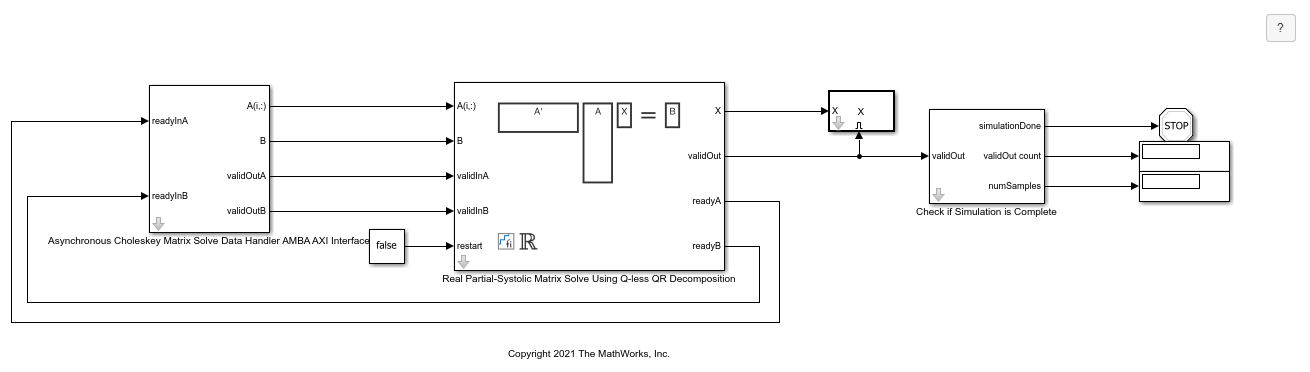# Implement Hardware-Efficient Real Partial-Systolic Matrix Solve Using Q-less QR Decomposition

This example shows how to implement a hardware-efficient solution to the real-valued matrix equation A'AX=B using the Real Partial-Systolic Matrix Solve Using Q-less QR Decomposition block.

### Define Matrix Dimensions

Specify the number of rows in matrix A, the number of columns in matrix A and rows in B, and the number of columns in matrix B.

```m = 300; % Number of rows in A n = 10; % Number of columns in A and rows in B p = 1; % Number of columns in B ```

### Generate Matrices

For this example, use the helper function `realRandomQlessQRMatrices` to generate random matrices A and B for the problem A'AX=B. The matrices are generated such that the elements of A and B are between -1 and +1, and A is full rank.

```rng('default') [A,B] = fixed.example.realRandomQlessQRMatrices(m,n,p); ```

### Select Fixed-Point Data Types

Use the helper function `realQlessQRMatrixSolveFixedpointTypes` to select fixed-point data types for input matrices A and B, and output X such that there is a low probability of overflow during the computation. For more information about how datatypes are selected, see the document FixedPointMatrixLibraryDatatypesExample.pdf in the current directory.

```max_abs_A = 1; % max(abs(A(:)) max_abs_B = 1; % max(abs(B(:)) f = 24; % Fraction length (bits of precision) T = fixed.example.realQlessQRMatrixSolveFixedpointTypes(m,n,max_abs_A,max_abs_B,f); A = cast(A,'like',T.A); B = cast(B,'like',T.B); OutputType = fixed.extractNumericType(T.X); ```

### Open the Model

```model = 'RealPartialSystolicQlessQRMatrixSolveModel'; open_system(model); ```The Data Handler subsystem in this model takes real matrices A and B as inputs. The `readyA` and `readyB` ports trigger the Data Handler. After sending a true validIn signal, there may be some delay before ready is set to false. When the Data Handler detects the leading edge of the `readyA` signal, the block sets `validInA` to true and sends the next row of A. When the Data Handler detects the leading edge of the `readyB` signal, the block sets `validInB` to true and sends the next matrix B. This protocol allows data to be sent whenever a leading edge of a ready signal is detected, ensuring that all data is processed.

### Set Variables in the Model Workspace

Use the helper function `setModelWorkspace` to add the variables defined above to the model workspace. These variables correspond to the block parameters for the Real Partial-Systolic Matrix Solve Using Q-less QR Decomposition block.

```numSamples = 1; % Number of samples fixed.example.setModelWorkspace(model,'A',A,'B',B,'m',m,'n',n,'p',p,... 'numSamples',numSamples,'OutputType',OutputType); ```

### Simulate the Model

```out = sim(model); ```

### Construct the Solution from the Output Data

The Real Partial-Systolic Matrix Solve Using Q-less QR Decomposition block outputs matrix X at each time step. When a valid result matrix is output, the block sets `validOut` to true.

```X = out.X; ```

### Verify the Accuracy of the Output

To evaluate the accuracy of the Real Partial-Systolic Matrix Solve Using Q-less QR Decomposition block, compute the relative error.

```relative_error = norm(double(A'*A*X - B))/norm(double(B)) %#ok<NOPTS> ```
```relative_error = 8.6289e-05 ```

## Support평가판 신청